# Chapter Notes: Motion Notes | Study Chapter Notes For Class 9 - Class 9

## Class 9: Chapter Notes: Motion Notes | Study Chapter Notes For Class 9 - Class 9

The document Chapter Notes: Motion Notes | Study Chapter Notes For Class 9 - Class 9 is a part of the Class 9 Course Chapter Notes For Class 9.
All you need of Class 9 at this link: Class 9

Introduction

• Rest: A body is said to be in a state of rest when its position does not change with respect to a reference point.
• Motion: A body is said to be in a state of motion when its position change continuously with reference to a point.
• Scalar quantity: It is the physical quantity having its own magnitude but no direction.
Example: Distance, Speed.
• Vector quantity: It is the physical quantity that requires both magnitude and direction.
Example: Displacement, Velocity.

Motion can be of different types depending upon the type of path by which the object is going through:

• Circulatory motion/Circular motion: In a circular path.
• Linear motion: In a straight-line path.
• Oscillatory/Vibratory motion: To and fro path with respect to origin.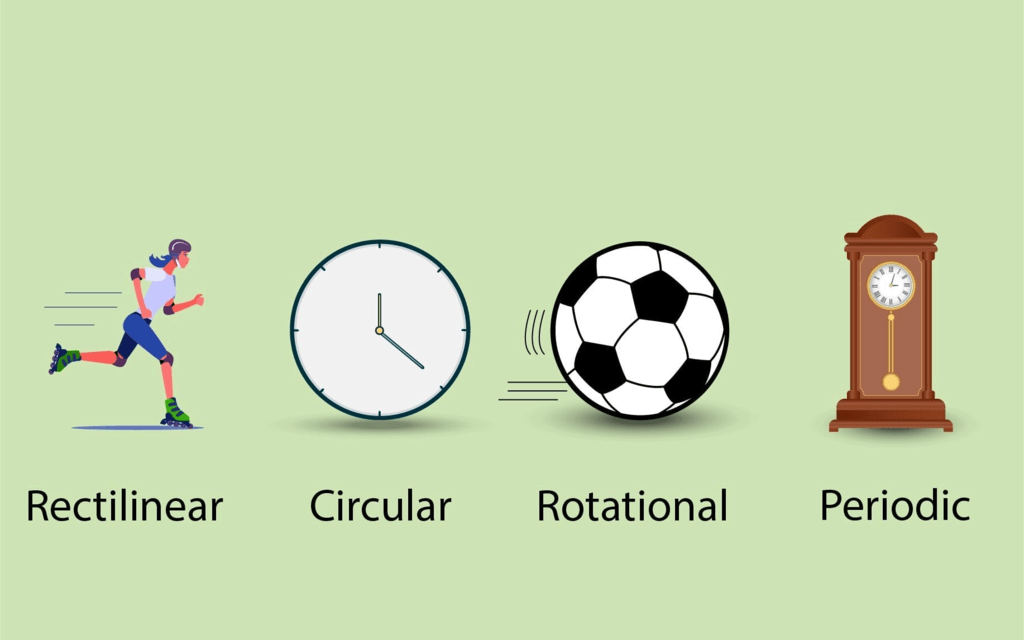Types of Motion

Distance and Displacement

1. Distance
• The actual path of length travelled by an object during its journey from its initial position to its final position is called the distance.
• Distance is a scalar quantity that requires only magnitude but no direction to explain it.
• Example: Ramesh travelled 65 km. (Distance is measured by odometer in vehicles.)
2. Displacement
• The shortest distance travelled by an object during its journey from its initial position to its final position is called displacement.
• Displacement is a vector quantity requiring both magnitude and direction for its explanation.
• Example: Ramesh travelled 65 km southwest from Clock Tower.
• Displacement can be zero (when the initial point and final point of motion are the same)
Example: Circular motion.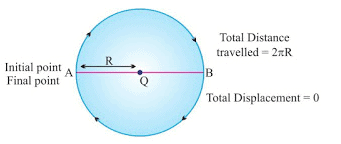3. Difference between Distance and Displacement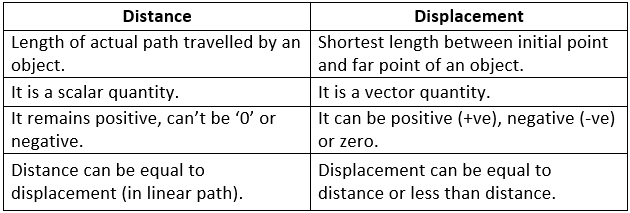Example 1: A body travels in a semicircular path of radius 10 m starting its motion from point ‘A’ to point ‘B’. Calculate the distance and displacement.
Sol. Given, π = 3.14, R = 10 m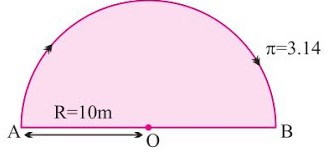Distance = πR = 3.14 × 10 = 31.4 m
Displacement = 2 × R = 2 × 10 = 20 m

Example 2: A body travels 4 km towards North then he turns to his right and travels another 4 km before coming to rest. Calculate
(i) total distance travelled,
(ii) total displacement.
Sol. Total distance travelled = OA + AB = 4 km + 4 km = 8 km
Total displacement = OB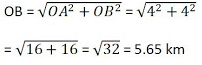Uniform and Non-uniform Motion

1. Uniform Motion
• When a body travels equal distances in equal intervals of time, then the motion is said to be a uniform motion.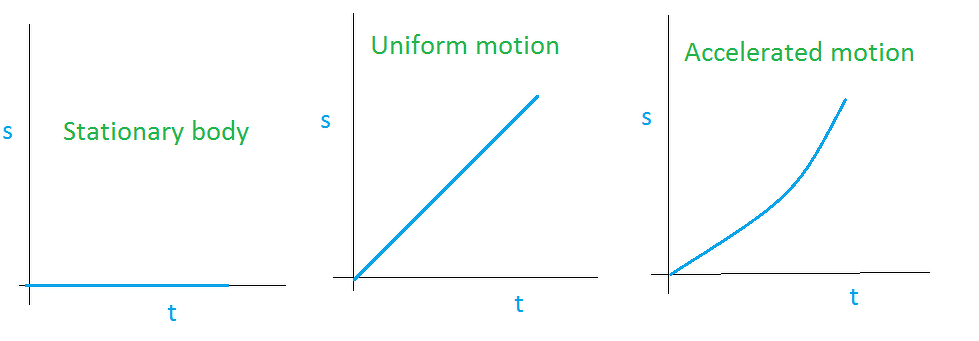2. Non-uniform Motion
• In this type of motion, the body will travel unequal distances in equal intervals of time.
• Two types of non-uniform-motion:
(i) Accelerated Motion: When a motion of a body increases with time.
(ii) De-accelerated Motion: When a motion of a body decreases with time.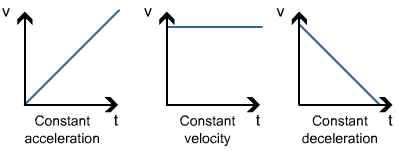Speed

• The measurement of distance travelled by a body per unit time is called speed.
i.e. Speed (v) = Distance Travelled/Time Taken = s/t
• SI unit: m/s (meters/second)
• If a body is executing uniform motion, then there will be a constant speed.
• If a body is travelling with non-uniform motion, then the speed will not remain uniform but have different values throughout the motion of such body.
• For non-uniform motion, the average speed will describe one single value of speed throughout the motion of the body.
i.e. Average speed = Total distance travelled/Total time taken

Conversion Factor
Change from km/hr to m/s = 1000m/(60×60)s = 5/18 m/s

Example: What will be the speed of body in m/s and km/hr if it travels 40 kms in 5 hrs?
Sol: Distance (s) = 40 km
Time (t)  = 5 hrs.
Speed (in km/hr) = Total distance/Total time = 40/5 = 8 km/hr
40 km = 40 × 1000 m = 40,000 m
5 hrs = 5 × 60 × 60 sec.
Speed (in m/s) = (40 × 1000)/(5×60 ×60) = 80/36 = 2.22 m/s

Velocity

• It is the speed of a body in a given direction.
• The measurement of displacement travelled by a body per unit time is called velocity.
i.e. Velocity = Displacement/Time
• SI unit of velocity: ms-1
• Velocity is a vector quantity. Its value changes when either its magnitude or direction changes.
• It can be positive (+ve), negative (-ve) or zero.
• For non-uniform motion in a given line, average velocity will be calculated in the same way as done in average speed.
i.e. Average velocity = Total displacement/Total time
• For uniformly changing velocity, the average velocity can be calculated as follows
Avg. Velocity (vavg) = (Initial velocity + Final velocity)/2 = (u+v)/2
where,  u = initial velocity, v = final velocity

Example 1: During first half of a journey by a body it travels with a speed of 40 km/hr and in the next half it travels with a speed of 20 km/hr. Calculate the average speed of the whole journey.
Sol: Speed during first half (v1) = 40 km/hr
Speed during second half (v2)  = 20 km/hr
Average speed = (v+ v2)/2 = (40 + 60)/2 = 60/2 = 30
Average speed by an object (body) = 30 km/hr.

Example 2: A car travels 20 km in first hour, 40 km in second hour and 30 km in third hour. Calculate the average speed of the train.
Sol: Speed in Ist hour = 20 km/hr
Distance travelled during 1st hr = 1 × 20= 20 km
Speed in 2nd hour = 40 km/hr
Distance travelled during 2nd hr = 1 × 40= 40 km
Speed in 3rd hour = 30 km/hr
Distance travelled during 3rd hr = 1 × 30= 30 km
Average speed = Total distance travelled/Total time taken
= (20 + 40 + 30)/3 = 90/3 = 30 km/hr

Acceleration

• Acceleration is seen in non-uniform motion and it can be defined as the rate of change of velocity with time.
i.e. Acceleration (a) = Change in velocity/Time = (v-u)/t
where, v = final velocity, u = initial velocity
• Here, v > u, then ‘a’ will be positive (+ve).

Example: A car speed increases from 40 km/hr to 60 km/hr in 5 sec. Calculate the acceleration of car.
Sol. u = 40km/hr = (40×5)/18 = 100/9 = 11.11 m/s
v  = 60 km/hr = (60×5)/18 = 150/9 = 16.66 m/s
t = 5 sec
a = (v-u)/t = (16.66 - 11.11)/5 = 5.55/5 = 1.11 ms-2

Retardation/Deceleration

• Deceleration is seen in non-uniform motion during decrease in velocity with time. It has same definition as acceleration.
i.e. Deceleration (a') = Change in velocity/Time = (v-u)/t
• Here, v < u, ‘a’ = negative (-ve).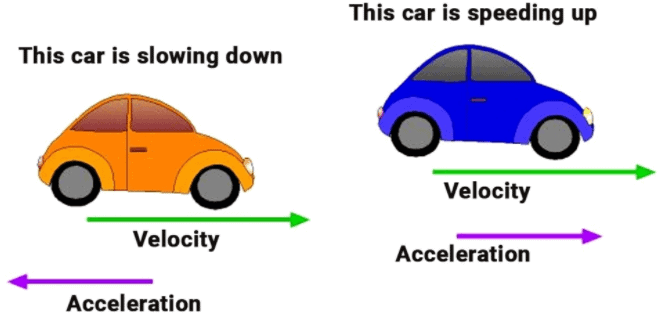Example: A car travelling with a speed of 20 km/hr comes into rest in 0.5 hrs. What will be the value of its retardation?
Sol. v = 0 km/hr, u = 20 km/hr, t = 0.5 hrs
Retardation, a = (v-u)/t = (0-20)/0.5 = -200/5 = -40 km hr-2

Graphical Representation of Equation

1. Distance-Time Graph (s/t graph)

(i) s/t graph for uniform motion: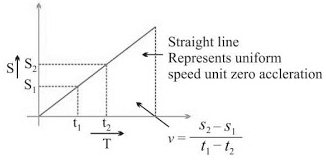(ii) s/t graph for non-uniform motion: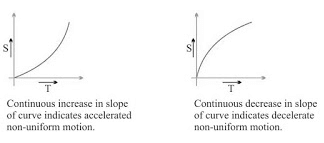(iii) s/t graph for a body at rest: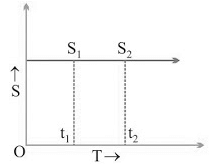v = (s2 - s1)/(t2 - t1)
But, s2 - s1
∴ v = 0/(t2 - t1) or v = 0

2. Velocity-Time Graph (v/t graph)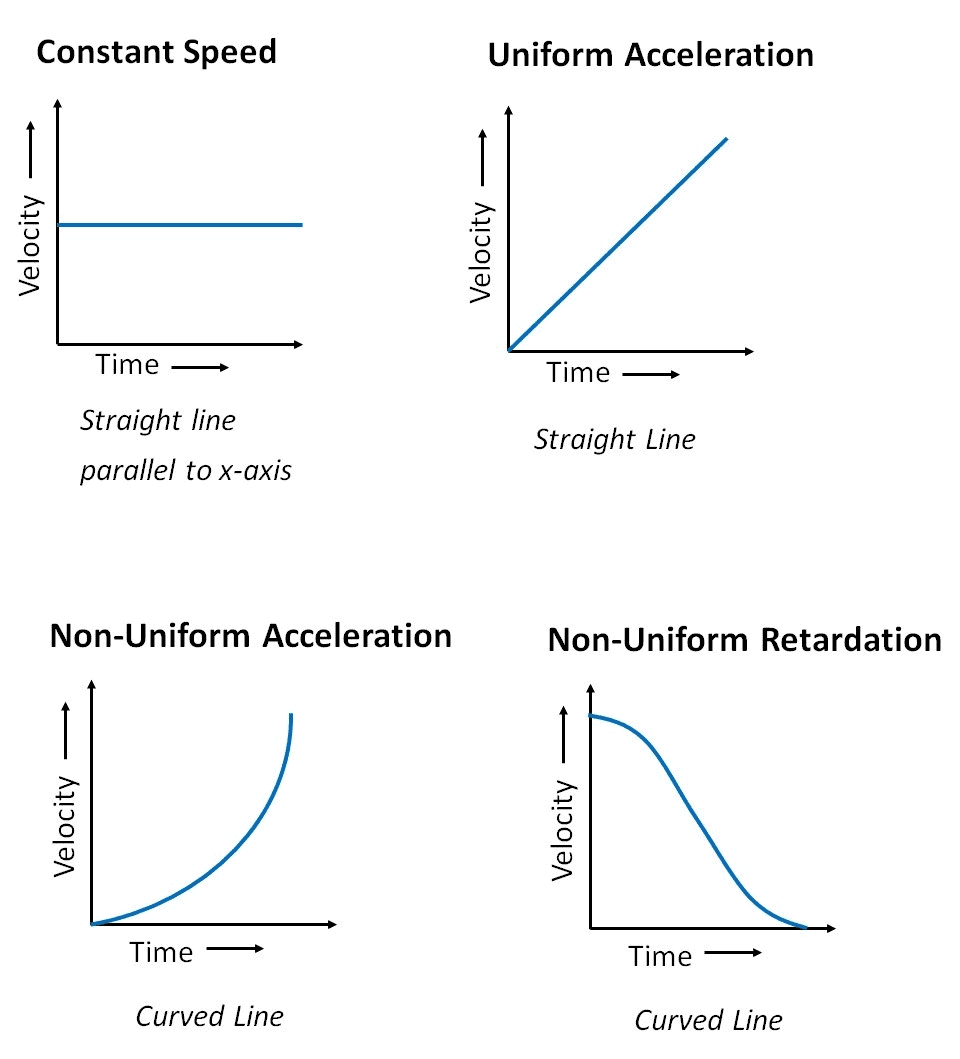(i) v/t graph for uniform motion: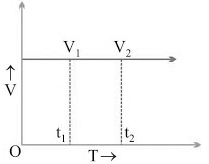a = (v2 - v1)/(t2 - t1)
But, v2 - v1
∴ a = 0/(t2 - t1) or a = 0

(ii) v/t graph for uniformly accelerated motion: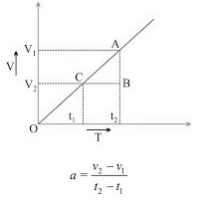In uniformly accelerated motion, there will be an equal increase in velocity in equal interval of time throughout the motion of body.

(iii) v/t graph for non-uniformly accelerated motion: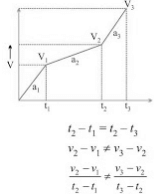a2 ≠ a1

(iv) v/t graph for uniformly decelerated motion: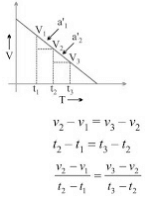a1' = a2'

(v) v/t graph for non-uniformly decelerated motion: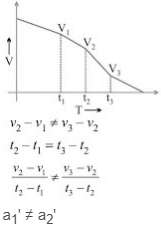Note: In v/t graph, the area enclosed between any two time intervals, t2 - t1, will represent the total displacement by that body.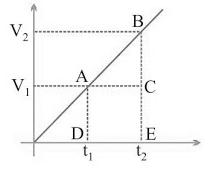Total distance travelled by body between t2 and t1
= Area of ∆ABC + Area of rectangle ACDB = ½ × (v2 – v1)×(t2 - t1) + v1× (t2 - t1)

Example: From the information given in the s/t graph, which of the following body ‘A’ or ‘B’ will be faster?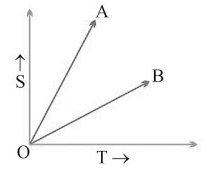Sol. vA > vB

Equation of Motion (For Uniformly Accelerated Motion)

1. First Equation: v = u + at

Final velocity = Initial velocity + Acceleration × Time
Graphical Derivation
Suppose a body has initial velocity ‘u’ (i.e., velocity at time t = 0 sec.) at point ‘A’ and this velocity changes to ‘v’ at point ‘B’ in ‘t’ secs. i.e., final velocity will be ‘v’.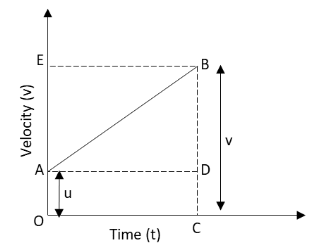For such a body there will be an acceleration.a = Change in velocity/Change in Time
⇒ a = (OB - OA)/(OC-0) = (v-u)/(t-0)
⇒ a = (v-u)/t
⇒ v = u + at

2. Second Equation: s = ut + ½ at2

Distance travelled by object = Area of OABC (trapezium)
= Area of OADC (rectangle) + Area of ∆ABD
= u × t + ½ × t × (v – u)
= ut + ½ × t × at
⇒ s = ut + ½ at2  (∵a = (v-u)/t)

3. Third Equation: v2 = u2 + 2as

s = Area of trapezium OABC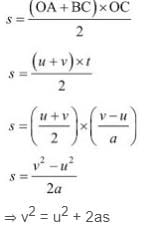Example 1: A car starting from rest moves with a uniform acceleration of 0.1 ms-2 for 4 mins. Find the speed and distance travelled.
Sol: u = 0 ms-1 (∵ car is at rest), a = 0.1 ms-2, t = 4 × 60 = 240 sec.
v = ?
From, v = u + at
v = 0 + (0.1 × 240) = 24 ms-1

Example 2: The brakes applied to a car produces deceleration of 6 ms -2 in opposite direction to the motion. If car requires 2 sec. to stop after application of brakes, calculate distance travelled by car during this time.
Sol: Deceleration, a = − 6 ms-2; Time, t = 2 sec.
Distance, s = ?
Final velocity, v = 0 ms-1 (∵ car comes to rest)
Now, v = u + at
⇒ u = v – at = 0 – (-6×2) = 12 ms-1
s = ut + ½ at= 12 × 2 + ½ (-6 × 22) = 24 – 12 = 12 m

Uniform Circular Motion

• If a body is moving in a circular path with uniform speed, then it is said to be executing the uniform circular motion.
• In such a motion the speed may be the same throughout the motion but its velocity (which is tangential) is different at each and every point of its motion. Thus, uniform circular motion is an accelerated motion.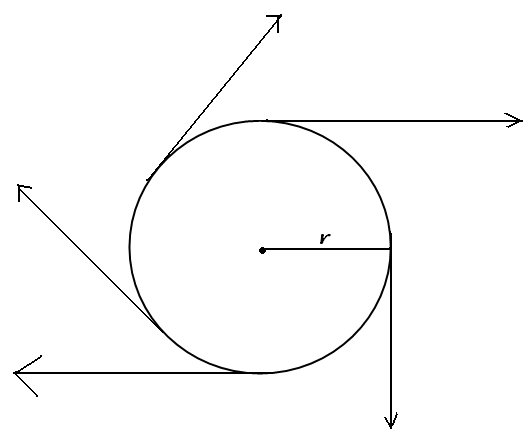Direction at different point while executing circular motion
The document Chapter Notes: Motion Notes | Study Chapter Notes For Class 9 - Class 9 is a part of the Class 9 Course Chapter Notes For Class 9.
All you need of Class 9 at this link: Class 9Use Code STAYHOME200 and get INR 200 additional OFF

## Chapter Notes For Class 9

215 docs

Track your progress, build streaks, highlight & save important lessons and more!

,

,

,

,

,

,

,

,

,

,

,

,

,

,

,

,

,

,

,

,

,

;